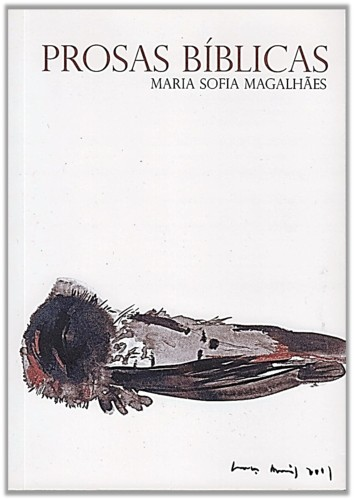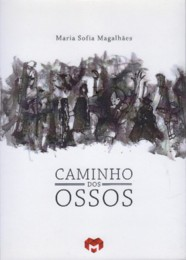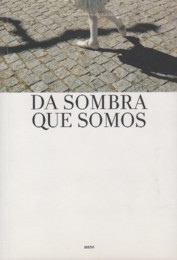Saltar para: Post , Comentários , Pesquisa e Arquivos 

## Um dia como os outros (28)

por Sofia Loureiro dos Santos, em 28.01.10

## 2 comentários### De hacker a 30.01.2010 às 19:52Quem me dera.

### Mais sobre mim### Subscrever por e-mail

A subscrição é anónima e gera, no máximo, um e-mail por dia.

1. 2022
2. J
3. F
4. M
5. A
6. M
7. J
8. J
9. A
10. S
11. O
12. N
13. D
14. 2021
15. J
16. F
17. M
18. A
19. M
20. J
21. J
22. A
23. S
24. O
25. N
26. D
27. 2020
28. J
29. F
30. M
31. A
32. M
33. J
34. J
35. A
36. S
37. O
38. N
39. D
40. 2019
41. J
42. F
43. M
44. A
45. M
46. J
47. J
48. A
49. S
50. O
51. N
52. D
53. 2018
54. J
55. F
56. M
57. A
58. M
59. J
60. J
61. A
62. S
63. O
64. N
65. D
66. 2017
67. J
68. F
69. M
70. A
71. M
72. J
73. J
74. A
75. S
76. O
77. N
78. D
79. 2016
80. J
81. F
82. M
83. A
84. M
85. J
86. J
87. A
88. S
89. O
90. N
91. D
92. 2015
93. J
94. F
95. M
96. A
97. M
98. J
99. J
100. A
101. S
102. O
103. N
104. D
105. 2014
106. J
107. F
108. M
109. A
110. M
111. J
112. J
113. A
114. S
115. O
116. N
117. D
118. 2013
119. J
120. F
121. M
122. A
123. M
124. J
125. J
126. A
127. S
128. O
129. N
130. D
131. 2012
132. J
133. F
134. M
135. A
136. M
137. J
138. J
139. A
140. S
141. O
142. N
143. D
144. 2011
145. J
146. F
147. M
148. A
149. M
150. J
151. J
152. A
153. S
154. O
155. N
156. D
157. 2010
158. J
159. F
160. M
161. A
162. M
163. J
164. J
165. A
166. S
167. O
168. N
169. D
170. 2009
171. J
172. F
173. M
174. A
175. M
176. J
177. J
178. A
179. S
180. O
181. N
182. D
183. 2008
184. J
185. F
186. M
187. A
188. M
189. J
190. J
191. A
192. S
193. O
194. N
195. D
196. 2007
197. J
198. F
199. M
200. A
201. M
202. J
203. J
204. A
205. S
206. O
207. N
208. D
209. 2006
210. J
211. F
212. M
213. A
214. M
215. J
216. J
217. A
218. S
219. O
220. N
221. D
222. 2005
223. J
224. F
225. M
226. A
227. M
228. J
229. J
230. A
231. S
232. O
233. N
234. D

### Maria Sofia MagalhãesÀ venda na livraria Ler DevagarÀ venda na Edita-me e na WookÀ venda na Derva Editores e na Wook